How to Do Physics Problems

Think of the paper on which you do the problem as a canvas and you are the artist.  Equations are beautiful structures and how you wield them is also an art.  If you start on a problem and part way through you see some previous steps that were unnecessary, write the problem over when you are finished so that the solution is as elegant as possible.  It will help you when studying because the solution will be easy to follow and it will make sense.

1.  Read the problem to get a general sense of it.

2.  Read it again, this time writing down all the assumptions and values given in the problem.

3.  Write one clear sentence describing the end goal of the problem.

4.  Draw a picture (even if you think you have the picture in your head, draw a picture anyway — you might be surprised at what more you will see).

5.  Define all variables.

6.  IMPORTANT!!!  Leave all the equations in terms of variables until the very end!!!

7.  Once you decide on your strategy, make a clear statement for each step you take.

8.  As you go, number equations you might refer back to later in the problem.

9.  If you use a result from earlier in the problem, refer back to it specifically, either by its number ("using (3) and (5) gives...") or by a statement which recalls your assumptions ("assuming the density is constant leads to...").

10.  Use recognized symbols for words whenever you can, for example:

 symbol definition example"is defined to be""implies"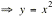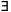"there exists"...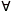"for all"..."increasing"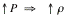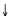"decreasing"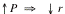"therefore"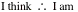11.  After you get an answer, check the units.

12.  If the answer is numerical, does the amount (and/or direction) make sense?

13.  If the answer is left in terms of the variables, does the relationship among the variables make sense?  (Here is the physics.)

14.  Box off the result.